# The OPTQP Procedure

### Example 12.1 Linear Least Squares Problem

The linear least squares problem arises in the context of determining a solution to an overdetermined set of linear equations. In practice, these equations could arise in data fitting and estimation problems. An overdetermined system of linear equations can be defined as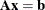where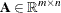,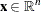,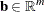, and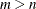. Since this system usually does not have a solution, you need to be satisfied with some sort of approximate solution. The most widely used approximation is the least squares solution, which minimizes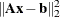.

This problem is called a least squares problem for the following reason. Let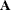,, and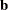be defined as previously. Let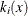be the kth component of the vector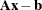: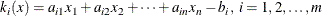By definition of the Euclidean norm, the objective function can be expressed as follows: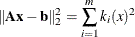Therefore, the function you minimize is the sum of squares of m terms; hence the term least squares. The following example is an illustration of the linear least squares problem; that is, each of the terms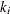is a linear function of x.function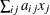plus a constant,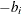.

Consider the following least squares problem defined by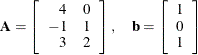This translates to the following set of linear equations: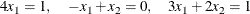The corresponding least squares problem is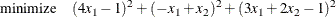The preceding objective function can be expanded to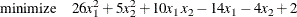In addition, you impose the following constraint so that the equation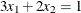is satisfied within a tolerance of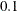: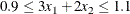You can create the QPS-format input data set by using the following SAS statements:

data lsdata;
input field1 $field2$ field3 $field4 field5$ field6 @;
datalines;
NAME     .      LEASTSQ     .            .         .
ROWS     .      .           .            .         .
N        OBJ    .           .            .         .
G        EQ3    .           .            .         .
COLUMNS  .      .           .            .         .
.        X1     OBJ        -14           EQ3       3
.        X2     OBJ        -4            EQ3       2
RHS      .      .           .            .         .
.        RHS    OBJ        -2            EQ3       0.9
RANGES   .      .           .            .         .
.        RNG    EQ3         0.2          .         .
BOUNDS   .      .           .            .         .
FR       BND1   X1          .            .         .
FR       BND1   X2          .            .         .
QUADOBJ  .      .           .            .         .
.        X1     X1          52           .         .
.        X1     X2          10           .         .
.        X2     X2          10           .         .
ENDATA   .      .           .            .         .
;


The decision variables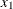and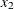are free, so they have bound type FR in the BOUNDS section of the QPS-format data set.

You can use the following SAS statements to solve the least squares problem:

proc optqp data=lsdata
printlevel = 0
primalout = lspout;
run;


The optimal solution is displayed in Output 12.1.1.

Output 12.1.1: Solution to the Least Squares Problem

 Primal Solution

Obs Objective
Function ID
RHS ID Variable
Name
Variable
Type
Linear Objective
Coefficient
Lower Bound Upper Bound Variable Value Variable
Status
1 OBJ RHS X1 F -14 -1.7977E308 1.7977E308 0.23810 O
2 OBJ RHS X2 F -4 -1.7977E308 1.7977E308 0.16190 O

The iteration log is shown in Output 12.1.2.

Output 12.1.2: Iteration Log

 NOTE: The problem LEASTSQ has 2 variables (2 free, 0 fixed). NOTE: The problem has 1 constraints (0 LE, 0 EQ, 0 GE, 1 range). NOTE: The problem has 2 constraint coefficients. NOTE: The objective function has 2 Hessian diagonal elements and 1 Hessian elements above the diagonal. NOTE: The QP presolver value AUTOMATIC is applied. NOTE: The QP presolver removed 0 variables and 0 constraints. NOTE: The QP presolver removed 0 constraint coefficients. NOTE: The presolved problem has 2 variables, 1 constraints, and 2 constraint coefficients. NOTE: The QP solver is called. NOTE: The Interior Point algorithm is used. NOTE: The deterministic parallel mode is enabled. NOTE: The Interior Point algorithm is using up to 4 threads. Primal        Bound         Dual Iter   Complement  Duality Gap       Infeas       Infeas       Infeas 0  1.91812E-02  5.89357E-03  1.96367E-08  0.00000E+00  3.53901E-04 1  9.04861E-04  2.83115E-04  7.25763E-10  0.00000E+00  1.30555E-05 2  1.53700E-05  4.94409E-06  7.77909E-12  0.00000E+00  1.30555E-07 3  1.53570E-07  4.93971E-08  8.81570E-14  0.00000E+00  1.30562E-09 NOTE: Optimal. NOTE: Objective = 0.0095238095. NOTE: The Interior Point solve time is 0.01 seconds. NOTE: The data set WORK.LSPOUT has 2 observations and 9 variables.